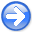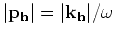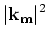Time-shift imaging condition in seismic migrationNext: Time-shift imaging condition Up: Angle transformation in wave-equation Previous: Angle transformation in wave-equation

## Space-shift imaging condition

Definingandas location and offset wavenumber vectors, and assuming, whereis the slowness at image locations, we can replaceandin equations (15)-(16):(17)(18)

Using the trigonometric identity(19)

we can eliminate from equations (17)-(18) the dependence on frequency and slowness, and obtain an angle decomposition formulation after imaging by expressingas a function of position and offset wavenumbers:(20)

We can construct angle-domain common-image gathers by transforming prestack migrated images using equation (20)(21)

In 2D, this transformation is equivalent with a slant-stack on migrated offset gathers. For 3D, this transformation is described in more detail by Fomel (2004) or Sava and Fomel (2005).Time-shift imaging condition in seismic migrationNext: Time-shift imaging condition Up: Angle transformation in wave-equation Previous: Angle transformation in wave-equation

2007-04-08